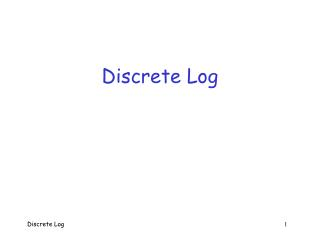# Discrete Log - PowerPoint PPT PresentationDownload PresentationDiscrete Log

Discrete LogDownload Presentation## Discrete Log

- - - - - - - - - - - - - - - - - - - - - - - - - - - E N D - - - - - - - - - - - - - - - - - - - - - - - - - - -
##### Presentation Transcript

1. Discrete Log Discrete Log 1

2. Discrete Logarithm • Discrete log problem: • Given p, g and ga (mod p), determine a • This would break Diffie-Hellman and ElGamal • Discrete log algorithms analogous to factoring, except no sieving • This makes discrete log harder to solve • Implies smaller numbers can be used for equivalent security, compared to factoring Discrete Log 2

3. Discrete Log Algorithms • We discuss three methods • Trial multiplication • Analogous to trial division for factoring • Baby-step giant-step • TMTO for trial multiplication • Index calculus • Analogous to Dixon’s algorithm Discrete Log 3

4. Trial Multiplication • The most obvious thing to do… • We know p, g and ga (mod p) • To find a, compute g2 (mod p), g3 (mod p), g4 (mod p), … • Until one matches ga (mod p) • Expected work is about p/2 Discrete Log 4

5. Baby Step Giant Step • Speed up to trial multiplication • Again, know p, g and x = ga (mod p) • We want to find exponent a • First, let m = sqrt(p  1) • Then a = im + j, some i,j  {0,1,…,m1} • How does this help? Next slide… Discrete Log 5

6. Baby Step Giant Step • Have x = ga (mod p)= gim+j (mod p) • Therefore, gj = xgim (mod p) • If we find i and j so that this holds, then we have found exponent a • Since a = im + j • How to find such i and j ? Discrete Log 6

7. Baby Step Giant Step • Algorithm: Given x = ga (mod p) • Giant steps: Compute and store in a table, xgim (mod p) for i = 0,1,…m1 • Baby steps: Compute gj (mod p) for j = 0,1,… until a match with table — obtain a = im + j • Expected work: sqrt(p) to compute table, sqrt(p)/2 to find j, for total of 1.5sqrt(p) • Storage: sqrt(p) required Discrete Log 7

8. Baby Step Giant Step Example • Spse g = 3, p = 101 and x = ga (mod p) = 37 • Then let m = 10 and compute giant steps: • Next, compute 3j (mod 101) until match found with last row • In this case, find 34 = 37320 (mod 101) • And we have found a = 24 Discrete Log 8

9. Index Calculus • Given p, g, x = ga (mod p), determine a • Analogous to Dixon’s algorithm • Except linear algebra phase comes first • Choose bound B and factor base • Suppose p0,p1,…,pn1 are primes in factor base • Precompute discrete logs: logg pi for each i • Can be done efficiently • Corresponds to linear algebra phase in Dixon’s Discrete Log 9

10. Index Calculus • Next, randomly select k  {0,1,2,…,p2} and compute y = xgk (mod p) until find y that factors completely over factor base • Then • Take logg and simplify to obtain a = logg x = (d0logg p0 + d0logg p0 + … + d0logg p0 k) (mod (p  1)) • And we have determined a • Note p  1 follows from Fermat’s Little Thm Discrete Log 10

11. Index Calculus Example • Spse: g = 3, p = 101, x = 3a = 94 (mod p) • We choose factor base 2,3,5,7 • Compute discrete logs: log32 = 29, log33 = 1, log35 = 96, log37 = 61 • Select random k, compute y = xgk (mod p) until y factors over factor base • For k = 10, find y = 50 = 252 mod (101) Discrete Log 11

12. Index Calculus Example • For k = 10, have y = 50 = 252 mod (101) • Then a = (log3 2 + 2 log3 5  10) (mod 100) = 29 + 296  10 = 11 (mod 100) • Easy to verify 311 = 94 (mod 101) • Work is same as Dixon’s algorithm • In particular, work is subexponential Discrete Log 12

13. Conclusions • Many parallels between factoring and discrete log algorithms • For example, Dixon’s and index calculus • For discrete log, not able to sieve • Therefore, no analog of quadratic sieve • For elliptic curve cryptosystems (ECC) • No analog of Dixon’s or index calculus… • …since no concept of a factor base • So ECC is secure with smaller parameters Discrete Log 13